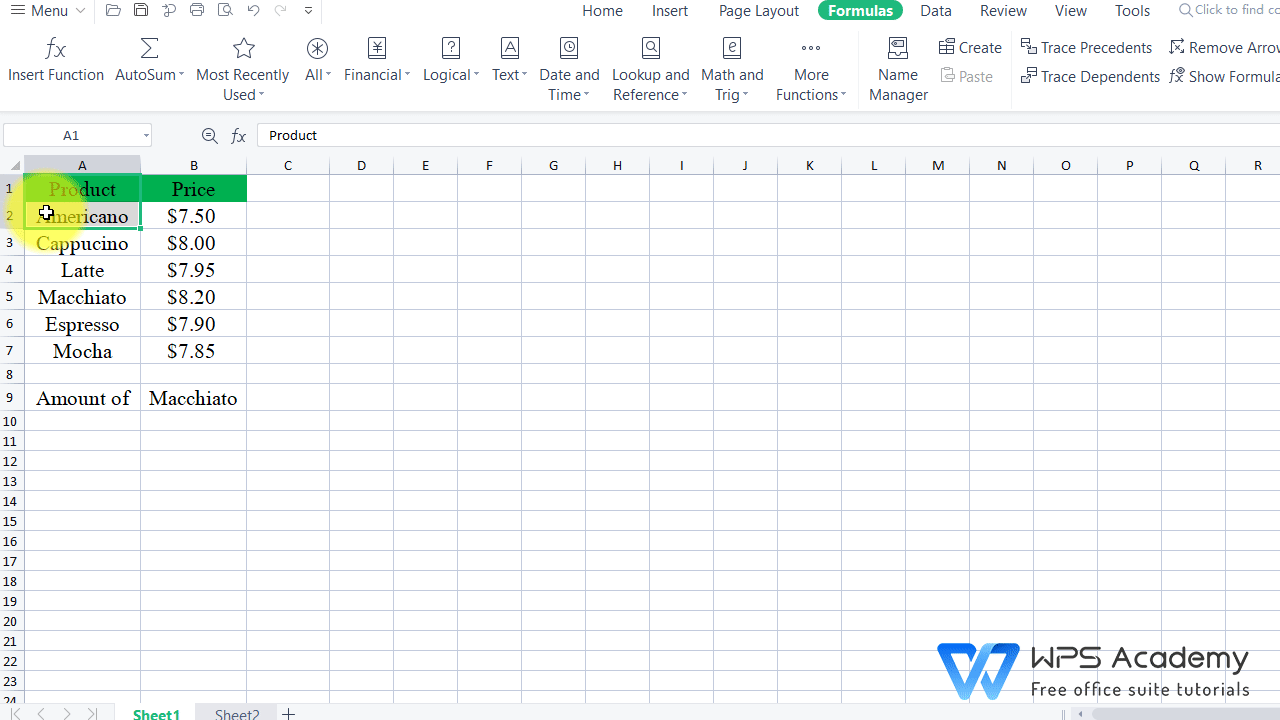# How to use the VLOOKUP function across multiple sheets in WPS Office Excel?

September 28, 2021
18.4K Views
0

A free Office suite fully compatible with Microsoft Office### Catalog

lIntroduction to VLOOKUP function.
lSteps to apply VLOOKUP formula across sheets in WPS Spreadsheet

## lIntroduction to VLOOKUP function.

VLOOKUP, respresenting Vertical Lookup, is a function used to look up data that meets the query conditionsin a table organized vertically. This formula returnsa value from a different column in the same row.It can also be used to look up values across sheets.

It's syntax is as follows:

=VLOOKUP (lookup_value, table_array, column_index_num, [range_lookup])

It includes 4 components:

Lookup_value: the value you want to look up

Table_array: the range where to look up

o Col_index_num: the order of the column where the data to be filled locates in

o Range_lookup: optional, FALSE or 0 (for exact match) / TRUE or 1 (for approximate match)

Notes:

o Vlookup function is only used for vertical data like this:o The lookup values to search must be in the first column of Table_array, like Americano, Cappucino and Latte in the table above.

## lSteps to apply VLOOKUP formula across sheets in WPS Spreadsheet

Take this worksheet as an example. In sheet 1, the data of product and price is given, while the data of sales amount are recorded in sheet 2. Assume that we need to look up the amount of Macchiato, and then we can follow the steps below.

1. Open the document in WPS Office. Click on the cell where you want to return the value. Click the shortcut Insert Function button, enter VLOOKUP in the pop-up dialog, and click OK.

2. In the pop-up dialog, enter B9 at Lookup_value, Sheet2!A2:B7 at Table_array, 2 at Col_index_num, and 0 at Range_lookup. In the formula,

=VLOOKUP(B9,sheet2!A2:B7,2,0)

o B9 is the value to search for;

o sheet 2!A2:B7 is the table from which to look up a value;

o 2 is the column number in the table from which to look up a value;

o 0 represents exact match.

And simply click OK to return the value.

3. Furthermore, if you want to look up more values with the same formula, enter \$ in corresponding places to lock the selected lookup range, so that we can drag the fill handle (+) to run the Auto Fill function without returning errors.

Thus, the formula is as follows:

=VLOOKUP(B9,Sheet2!\$A\$2:\$B\$7,2,0)